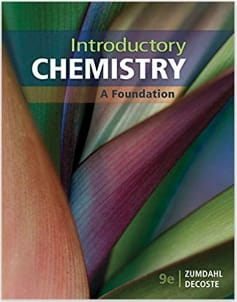Chemistry Introductory Chemistry# Introductory Chemistry Zumdahl & DeCoste • 9th Edition • 978-1337399425

Not the textbook you were looking for? Pick another one here.

## Ch.1 - Chemistry: An Introduction

Laboratory Materials Experimental Error

## BONUS: Mathematical Operations and Functions

Multiplication and Division Operations Addition and Subtraction Operations Power and Root Functions Power and Root Functions The Quadratic Formula

## Ch.5 - Nomenclature

Naming Ionic Compounds Writing Ionic Compounds Naming Ionic Hydrates Naming Acids Naming Molecular Compounds Functional Groups in Chemistry

## Ch.6 - Chemical Reactions: An Introduction

Balancing Chemical Equations

## Ch.8 - Chemical Composition

Empirical Formula Molecular Formula Combustion Analysis Combustion Apparatus Mass Percent

## Ch.9 - Chemical Quantities

Calculating Molar Mass Mole Concept Stoichiometry Limiting Reagent Percent Yield

## Ch.12 - Chemical Bonding Pt. 2

Electron Geometry Bond Angles Hybridization Molecular Polarity

## Ch.14 - Liquids and Solids

Intermolecular Forces Phase Diagram Heating and Cooling Curves Clausius-Clapeyron Equation Unit Cell Molecular Orbital Theory MO Theory: Heteronuclear Diatomic Molecules

## Ch.15 - Solutions

Solutions, Molarity and Intermolecular Forces Calculate Molarity Molality Mass Percent Mole Fraction Henry's Law

## Ch.17 -Equilibrium

Chemical Equilibrium ICE Chart Equilibrium Expressions Le Chatelier's Principle The Reaction Quotient Ksp

## Ch.18 - Oxidation-Reduction Reactions and Electrochemistry

Redox Reaction Balancing Redox Reaction The Nernst Equation Faraday's Constant Galvanic Cell Batteries and Electricity

## Ch.19 - Radioactivity and Nuclear Energy

Radioactive Decay Band of Stability Magic Numbers First Order Half Life